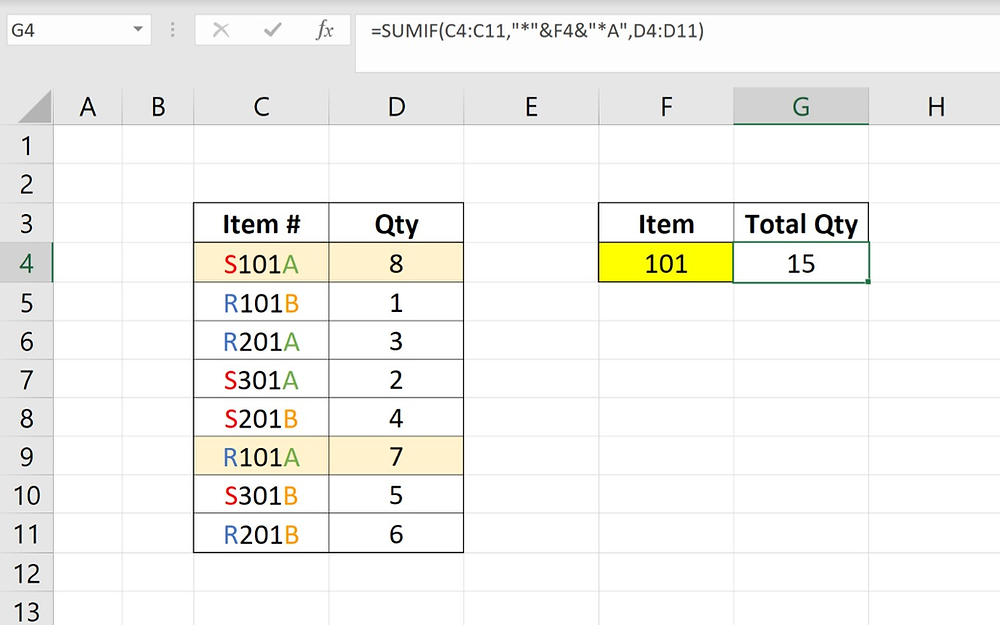Search
• Skylar

# How to Sum Values If a Cell Contains a Specific Text

In this video we will be showing you how to sum values if a cell contains a specific text. Additionally, we will reveal how to enhance the SUMIF function to create criteria that matches values beginning or ending with specific text.If we look at this table here, we have item numbers in one column and quantity in another. Our goal is to sum the quantities depending on the text that the item numbers contain. For example, if we select 101 from the drop down list, we want a formula to output the sum of every item that contains the string "101". We will be using the SUMIF function to make this possible.

String: also know as text, is a group of characters that are used as data.

## Syntax

=SUMIF(range, criteria, [sum_range])

## Arguments

• Range - is the range of cells you want evaluated.

• Criteria - the criteria used to determine which cells to add.

• Sum_range - are the actual cells to sum. If omitted, the cells in range are used.

## Notes:

The asterisk marks (*) are considered wildcards, meaning that it can match any sequence of characters.

The ampersand signs (&) are meant for combining the wildcards with the root criteria.

## Examples:

Let's say we want to sum the quantities for the item numbers that start with the letter "R" and contains "201", we have to add a "R" in our formula before the first asterisk mark.

=SUMIF(C4:C11,"*R"&F4&"*",D4:D11)Now we get an output of 9 because we have one R201 with a quantity of 3 and another with a quantity of 6.

Same goes for summing quantities for item numbers that end with a specific letter.

=SUMIF(C4:C11,"*"&F4&"*A",D4:D11)

• Formula for item numbers containing "101" and end with "A"Another option is to remove F4 completely and sum the quantities for item numbers that start and end with a specific character.

=SUMIF(C4:C11,"*S"&"*B",D4:D11)

• Formula for item numbers that start with "S" and end with "B".